Documentation

Modal Analysis of a Flexible Flying Wing Aircraft

This example shows computation of bending modes of a flexible wing aircraft. The vibration response of the wing is collected at multiple points along its span. The data is used to identify a dynamic model of the system. The modal parameters are extracted from the identified model. The modal parameter data is combined with the sensor position information to visualize the various bending modes of the wing. This example requires Signal Processing Toolbox™.

The Flexible Wing Aircraft

In this example, we study the data gathered from a small flexible flying wing aircraft built at the Uninhabited Aerial Vehicle Laboratories, University of Minnesota . The geometry of the aircraft is shown below.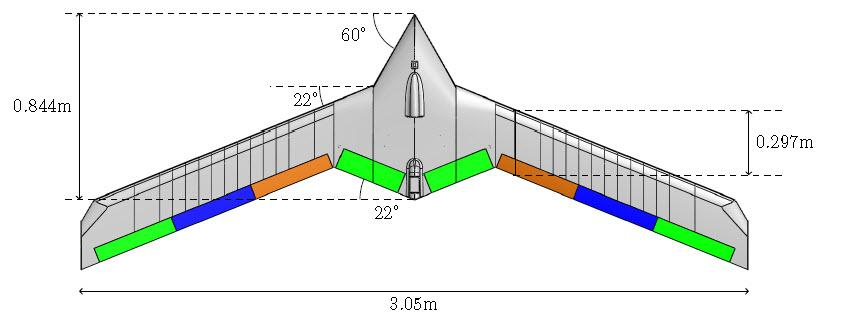The aircraft wing can undergo large deformations under loading. The flexible mode frequencies are lower than those in common aircraft with more rigid wings. This flexible design reduces material costs, increases agility and the flight range of the aircraft. However, if not controlled, the flexible modes can lead to catastrophic aeroelastic instabilities (flutter). Designing effective control laws for suppressing these instabilities requires accurate determination of the wing's various bending modes.

Experimental Setup

The objective of the experiment is to gather vibration response of the aircraft at various locations in response to an external excitation. The aircraft is suspended from a wooden frame using a single spring at its center of gravity. The spring is sufficiently flexible so that the natural frequency of the spring-mass oscillation does not interfere with the fundamental frequencies of the aircraft. An input force is applied via an Unholtz-Dickie Model 20 electrodynamic shaker near the center of the aircraft.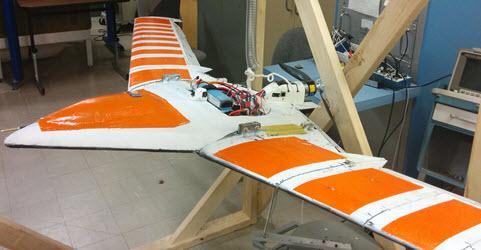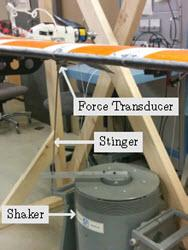Twenty PCB-353B16 accelerometers are placed along the wing span to collect the vibration response as shown in the next figure.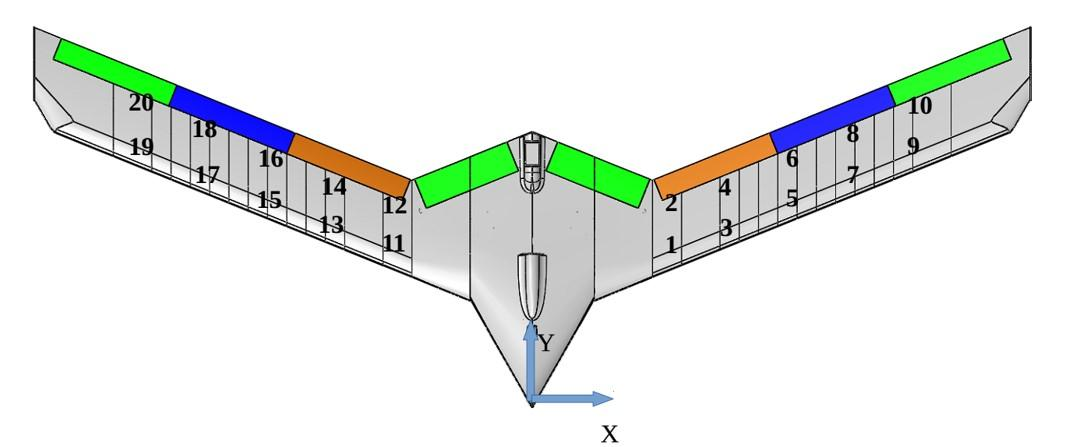The shaker input command is specified as a constant amplitude chirp input of the form $\mathit{A}\text{\hspace{0.17em}}\mathrm{sin}\left(\omega \left(\mathit{t}\right)\mathit{t}\right)$. The chirp frequency varies linearly with time, that is, $\omega \left(\mathit{t}\right)={\mathit{c}}_{0}+{\mathit{c}}_{1}\mathit{t}$. The frequency range covered by the input signal is 3–35 Hz. The data is collected by two accelerometers (leading and trailing edge accelerometers at one x-location) at a time. Hence 10 experiments are conducted to collect all the 20 accelerometer responses. The accelerometer and force transducer measurements are all sampled at 2000 Hz.

Data Preparation

The data is represented by 10 sets of two-output/one-input signals, each containing 600K samples. Load the data from MAT file FlexibleWingData.mat:

The variable MeasuredData is a structure with fields Exp1, Exp2, ..., Exp10. Each field is a structure with fields y and u containing the two responses and the corresponding input force data. Plot the data for the first experiment.

fs = 2000;                % data sampling frequency
Ts = 1/fs;                % sample time
y = MeasuredData.Exp1.y;  % output data (2 columns, one for each accelerometer)
u = MeasuredData.Exp1.u;  % input force data
t = (0:length(u)-1)' * Ts;
figure
subplot(211)
plot(t,y)
ylabel('Outputs (m/s^2)')
subplot(212)
plot(t,u)
ylabel('Input')
xlabel('Time (seconds)')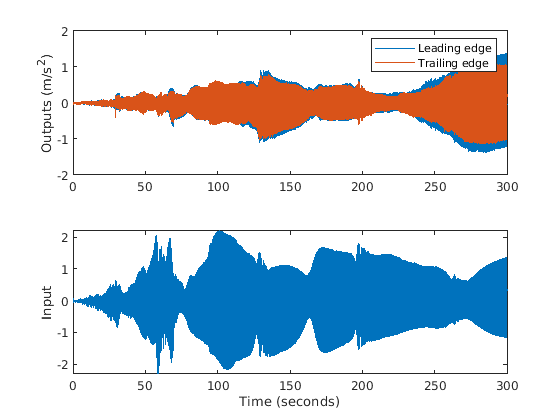In order to prepare data for model identification, the data is packaged into iddata objects. The iddata objects are standard way of packaging time-domain data in System Identification Toolbox™. The input signal is treated as bandlimited.

ExpNames = fieldnames(MeasuredData);
Data = cell(1, 10);
for k = 1:10
y =  MeasuredData.(ExpNames{k}).y;
u =  MeasuredData.(ExpNames{k}).u;
Data{k} = iddata(y, u, Ts, 'InterSample', 'bl');
end

Merge the dataset objects into one multi-experiment data object.

Data = merge(Data{:})
Data =

Time domain data set containing 10 experiments.

Experiment   Samples      Sample Time
Exp1      600001            0.0005
Exp2      600001            0.0005
Exp3      600001            0.0005
Exp4      600001            0.0005
Exp5      600001            0.0005
Exp6      600001            0.0005
Exp7      600001            0.0005
Exp8      600001            0.0005
Exp9      600001            0.0005
Exp10     600001            0.0005

Outputs      Unit (if specified)
y1
y2

Inputs       Unit (if specified)
u1

Model Identification

We want to identify a dynamic model whose frequency response matches that of the actual aircraft as closely as possible. A dynamic model encapsulates a mathematical relationship between the inputs and outputs of the system as a differential or difference equation. Examples of dynamic models are transfer functions and state-space models. In System Identification Toolbox, dynamic models are encapsulated by objects such as idtf (for transfer functions), idpoly (for AR, ARMA models) and idss (for state-space models). Dynamic models can be created by running estimation commands such as tfest and ssest commands on measured data in either time-domain or frequency-domain.

For this example, we first convert the measured time-domain data into frequency response data by empirical transfer function estimation using the etfe command. The estimated FRF is then used to identify a state-space model of the aircraft's vibration response. It is possible to directly use time-domain data for dynamic model identification. However, FRF form of data allows compression of large datasets into fewer samples as well as more easily adjust estimation behavior to relevant frequency ranges. FRFs are encapsulated by idfrd objects.

Estimate a two-output/one-input frequency response function (FRF) for each data experiment. Use no windowing. Use 24,000 frequency points for response computation.

G = cell(1, 10);
N = 24000;
for k = 1:10
% Convert time-domain data into a FRF using ETFE command
Data_k = getexp(Data, k);
G{k} = etfe(Data_k, [], N); % G{k} is an @idfrd object
end

Concatenate all FRFs into a single 20-output/one-input FRF.

G = cat(1, G{:});     % concatenate outputs of all estimated FRFs
G.OutputName = 'y';   % name outputs 'y1', 'y2', ..., 'y20'
G.InterSample = 'bl';

To get a feel for the estimated frequency response, plot the amplitude for outputs 1 and 15 (picked arbitrarily). Zoom into the frequency range of interest (4–35 Hz).

opt = bodeoptions;           % plot options
opt.FreqUnits = 'Hz';        % show frequency in Hz
opt.PhaseVisible = 'off';    % do not show phase
OutputNum = [1 15];          % pick outputs 1 and 15 for plotting
clf
bodeplot(G(OutputNum, :), opt)   % plot frequency response
xlim([4 35])
grid onThe FRF shows at least 9 resonant frequencies. For analysis we want to focus on 6-35 Hz frequency span where the most critical flexible bending modes of the aircraft lie. Hence reduce the FRF to this frequency region.

f = G.Frequency/2/pi;           % extract frequency vector in Hz (G stores frequency in rad/s)
Gs = fselect(G, f>6 & f<=32)    % "fselect" selects the FRF in the requested range (6.5 - 35 Hz)
Gs =
IDFRD model.
Contains Frequency Response Data for 20 output(s) and 1 input(s).
Response data is available at 624 frequency points, ranging from 37.96 rad/s to 201.1 rad/s.

Sample time: 0.0005 seconds
Output channels: 'y(1)', 'y(2)', 'y(3)', 'y(4)', 'y(5)', 'y(6)', 'y(7)', 'y(8)', 'y(9)', 'y(10)', 'y(11)', 'y(12)', 'y(13)', 'y(14)', 'y(15)', 'y(16)', 'y(17)', 'y(18)', 'y(19)', 'y(20)'
Input channels: 'u1'
Status:
Estimated model

Gs thus contains the frequency response measurements at the 20 measurement locations. Next, identify a state-space model to fit Gs. The subspace estimator n4sid provides a quick noniterative estimate. The state-space model structure is configured as follows:

1. We estimate an 18th-order continuous-time model. The order was found after some trials with various orders and checking the resulting fit of the model to the FRF.

2. The model contains a feedthrough term (D matrix is non-zero). This is because we are limiting our analysis to $\le$ 35 Hz while the wing's bandwidth is significantly larger than that (response is significant at 35 Hz).

3. To speed up computation, we suppress computation of parameter covariance.

4. The FRF magnitude varies significantly across the frequency range. In order to ensure that the low amplitudes receive equal emphasis as the higher amplitudes, we apply a custom weighting that varies inversely as the square root of the 11th response. The choice of 11th output is somewhat arbitrary but works since all 20 FRFs have similar profiles.

We set up the estimation options for n4sid using n4sidOptions.

FRF = squeeze(Gs.ResponseData);
Weighting = mean(1./sqrt(abs(FRF))).';
n4Opt = n4sidOptions('EstimateCovariance',false,...
'WeightingFilter',Weighting,...
'OutputWeight',eye(20));
sys1 = n4sid(Gs,24,'Ts',0,'Feedthrough',true,n4Opt);
Fit = sys1.Report.Fit.FitPercent'
Fit = 1×20

57.0200   57.9879   85.0160   86.3815   80.4879   80.4430   58.2216   45.2692   61.5057   76.7612   84.7305   86.2600   86.4266   85.0251   76.9208   82.1191   74.7982   79.6837   67.9078   76.7249

The "Fit" numbers shows the percentage fit between the data (Gs) and model's (sys1) frequency response using a normalized root-mean-square-error (NRMSE) goodness-of-fit measure. The poorest and best fits are plotted next.

[~,Imin] = min(Fit);
[~,Imax] = max(Fit);
clf
bodeplot(Gs([Imin, Imax],:), sys1([Imin, Imax],:), opt);
xlim([6 32])
title('Worst (top) and best (bottom) fits between data and model')
grid on
legend('Data', 'Model')The fits achieved with model sys1 can be improved even more by iterative nonlinear least-squares refinement of model's parameters. This can be achieved using the ssest command. We set up the estimation options for ssest using the ssestOptions command. This time the parameter covariance is also estimated.

ssOpt = ssestOptions('EstimateCovariance',true,...
'WeightingFilter',n4Opt.WeightingFilter,...
'SearchMethod','lm',...     % use Levenberg-Marquardt search method
'Display','on',...
'OutputWeight',eye(20));
sys2 = ssest(Gs, sys1, ssOpt);  % estimate state-space model (this takes several minutes)
Fit = sys2.Report.Fit.FitPercent'
Fit = 1×20

89.7225   89.5185   89.7260   90.4986   88.5522   88.8727   81.3225   83.5975   75.9215   83.1763   91.1358   89.7310   90.6844   89.8444   89.6685   89.1467   87.8532   88.0385   84.2898   83.3578

As before we plot the worst and the best fits. We also visualize the 1-sd confidence region for the model's frequency response.

[~, Imin] = min(Fit);
[~, Imax] = max(Fit);
clf
h = bodeplot(Gs([Imin, Imax],:), sys2([Imin, Imax],:), opt);
showConfidence(h, 1)
xlim([6.5 35])
title('Worst (top) and best (bottom) fits between data and refined model')
grid on
legend('Data', 'Model (sys2)')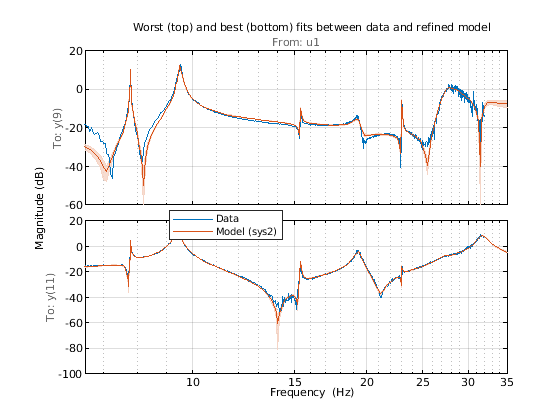The refined state-space model sys2 fits the FRFs quite well in the 7–20 Hz region. The uncertainty bounds are tight around most resonant locations. We estimated a 24th-order model which means that there are at most 12 oscillatory modes in the system sys2. Use the modalfit command to fetch the natural frequencies in Hz for these modes.

f = Gs.Frequency/2/pi;     % data frequencies (Hz)
fn = modalfit(sys2, f, 12); % natural frequencies (Hz)
disp(fn)
7.7721
7.7953
9.3147
9.4009
9.4910
15.3463
19.3291
23.0219
27.4164
28.7256
31.7014
63.3034

The values in fn suggest two frequencies very close to 7.8 Hz and three close to 9.4 Hz. An inspection of frequency responses near these frequencies reveals that the peaks location shift a little bit across outputs. These discrepancies may be removed by better control over the experiment process, performing direct time-domain identification with input bandwidth limited to narrow range centered at these frequencies, or fitting a single oscillatory mode to the frequency response around these frequencies. These alternatives are not explored in this example.

Modal Parameter Computation

We can now use the model sys2 to extract the modal parameters. An inspection of the FRFs indicates around 10 modal frequencies, roughly around the frequencies [5 7 10 15 17 23 27 30] Hz. We can make this assessment more concrete by using the modalsd command that checks the stability of modal parameters as the order of the underlying model is changed. This operation takes a long time. Hence the resulting plot is inserted directly as an image. Execute the code inside the comment block below to reproduce the figure.

FRF = permute(Gs.ResponseData,[3 1 2]);
f = Gs.Frequency/2/pi;
%{
figure
pf = modalsd(FRF,f,fs,'MaxModes',20,'FitMethod','lsrf');
%}Inspection of the plot and pf values suggests a refined list of true natural frequencies:

Freq = [7.8 9.4 15.3 19.3 23.0 27.3 29.2 31.7];

We use the values in Freq vector as a guide for picking most dominant modes from the model sys2. This is done using the modalfit command.

[fn,dr,ms] = modalfit(sys2,f,length(Freq),'PhysFreq',Freq);

fn are the natural frequencies (in Hz), dr the corresponding damping coefficients and ms the normalized residuals as column vectors (one column for each natural frequency). In the process of extraction of these modal parameters, only the stable, under-damped poles of the model are used. The ms columns contain data for only the poles with positive imaginary part.

Mode Shape Visualization

To visualize the various bending modes, we need the modal parameters extracted above. In addition we need information on the location of the measurement points. These positions (x-y values) is recorded for each accelerometer in the matrix AccePos:

AccelPos = [...   % see figure 2
16.63 18.48;   % nearest right of center
16.63 24.48;
27.90 22.22;
27.90 28.22;
37.17 25.97;
37.17 31.97;
46.44 29.71;
46.44 35.71;
55.71 33.46;
55.71 39.46;  % farthest right
-16.63 18.48; % nearest left of center
-16.63 24.18;
-27.90 22.22;
-27.90 28.22;
-37.17 25.97;
-37.17 31.97;
-46.44 29.71;
-46.44 35.71;
-55.71 33.46;
-55.71 39.46]; % farthest left

The mode shapes are contained in the matrix ms where each column corresponds to the shape for one frequency. Animate the mode by superimposing mode shape amplitudes over the sensor coordinates and varying the amplitudes at the mode's natural frequency. The animation shows the bending with no damping. As an example, consider the mode at 15.3 Hz.

AnimateOneMode(3, fn, ms, AccelPos);Conclusions

This example shows a parametric model identification based approach to modal parameter estimation. Using a state-space model of 24th order, 8 stable oscillatory modes in the frequency region 6–32 Hz were extracted. The modal information was combined with the knowledge of the accelerometer positions to visualize the various bending modes. Some of these modes are shown in the figures below.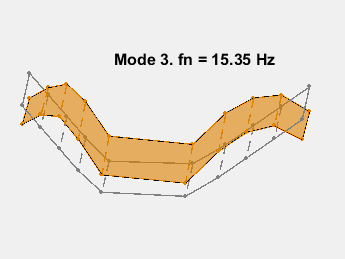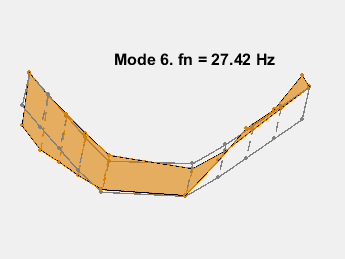References

 Gupta, Abhineet, Peter J. Seiler, and Brian P. Danowsky. "Ground Vibration Tests on a Flexible Flying Wing Aircraft." AIAA Atmospheric Flight Mechanics Conference, AIAA SciTech Forum. (AIAA 2016-1753).

function AnimateOneMode(ModeNum, fn, ModeShapes, AccelPos)
% Animate one mode.
% ModeNum: Index of the mode.

% Reorder the sensor locations for plotting so that a continuous,
% non-intersecting curve is traced around the body of the aircraft.
PlotOrder = [19:-2:11, 1:2:9, 10:-2:2, 12:2:20, 19];
Fwd = PlotOrder(1:10);
Aft = PlotOrder(20:-1:11);
x = AccelPos(PlotOrder,1);
y = AccelPos(PlotOrder,2);
xAft = AccelPos(Aft,1);
yAft = AccelPos(Aft,2);
xFwd = AccelPos(Fwd,1);
yFwd = AccelPos(Fwd,2);

wn = fn(ModeNum)*2*pi;  % Mode frequency in rad/sec
T = 1/fn(ModeNum);      % Period of modal oscillation
Np  = 2.25;             % Number of periods to simulate
tmax = Np*T;            % Simulate Np periods
Nt = 100;               % Number of time steps for animation
t = linspace(0,tmax,Nt);
ThisMode = ModeShapes(:,ModeNum)/max(abs(ModeShapes(:,ModeNum))); % normalized for plotting
z0 = ThisMode(PlotOrder);
zFwd = ThisMode(Fwd);
zAft = ThisMode(Aft);

clf
col1 = [1 1 1]*0.5;
xx = reshape([[xAft, xFwd]'; NaN(2,10)],[2 20]);
yy = reshape([[yAft, yFwd]'; NaN(2,10)],[2 20]);
plot3(x,y,0*z0,'-', x,y,0*z0,'.', xx(:), yy(:), zeros(40,1),'--',...
'Color',col1,'LineWidth',1,'MarkerSize',10,'MarkerEdgeColor',col1);
xlabel('x')
ylabel('y')
zlabel('Amplitude')
ht = max(abs(z0));
axis([-100  100  10  40 -ht ht])
view(5,55)
title(sprintf('Mode %d. FN = %s Hz', ModeNum, num2str(fn(ModeNum),4)));

% Animate by updating z-coordinates.
hold on
col2 = [0.87 0.5 0];
h1 = plot3(x,y,0*z0,'-', x,y,0*z0,'.', xx(:), yy(:), zeros(40,1),'--',...
'Color',col2,'LineWidth',1,'MarkerSize',10,'MarkerEdgeColor',col2);
h2 = fill3(x,y,0*z0,col2,'FaceAlpha',0.6);
hold off

for k = 1:Nt
Rot1 = cos(wn*t(k));
Rot2 = sin(wn*t(k));
z_t = real(z0)*Rot1 - imag(z0)*Rot2;
zAft_t = real(zAft)*Rot1 - imag(zAft)*Rot2;
zFwd_t = real(zFwd)*Rot1 - imag(zFwd)*Rot2;
zz = reshape([[zAft_t, zFwd_t]'; NaN(2,10)],[2 20]);
set(h1(1),'ZData',z_t)
set(h1(2),'ZData',z_t)
set(h1(3),'ZData',zz(:))
h2.Vertices(:,3) = z_t;
pause(0.1)
end

end
System Identification Toolbox DocumentationGet trial now